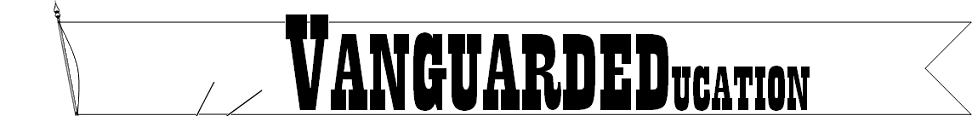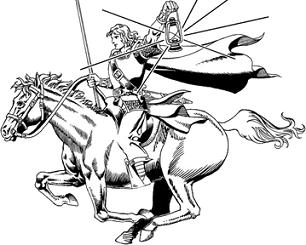•• Home
•• Curriculum
• Shopping
•• Free Items
••• Contact
•How to Begin the Mathematics Course

Vanguard Education recommends that a student begin formal mathematics class on the sixth birthday. This can be delayed until later in the sixth year, or even to the seventh birthday, if the student is not ready at age six.
What follows are recommendations of Vanguard Education. These recommendations should be altered to fit each individual student.
Begin with Saxon 3. This is a United States grade 3 (Commonwealth year 5) mathematics book. Vanguard Education recommends that Saxon 1 and 2 not be used.
The Saxon 3 lessons are divided into part A and part B. On the first day of mathematics class, the student does all of Lesson 1.
Beginning on the second day of class, the student does one numbered lesson over two days’ time. For the first day of a lesson, do the math meeting and the lesson. On the second day, the student completes sides A and B of the worksheet. At this pace, it will take the student more than a year to complete Saxon 3.
Saxon 3 has tests. If there is a test and a worksheet (side A and B) in the same lesson, do the test and the math meeting the first day, and do the lesson and worksheet (A and B) on the second day. If there is a test and no worksheet for the lesson, then do the math meeting and then the test on the first day. The second day is for corrections on the test and teaching the lesson.
When the student completes Saxon 3, the student begins Saxon 54 on the following day. Begin with whole lessons daily. If the child becomes bogged down and spends excessive time on math, switch to half lessons as described below.
Flash cards are cards with simple addition, subtraction, multiplication, and division problems on them. The purpose of flash cards is to assist the student in memorizing simple mathematics problems, such as 2+3= , 7-3= , 5x5= , 36÷6= . Flash cards are used in the Saxon lessons. The book tells when to use them.

### HOW TO TEACH

The Saxon 3 book gives instructions to the teacher on what to do for each lesson. Younger students will require larger amounts of interaction with the teacher. Plan on sitting and teaching the entire lesson to the child. Then let the child do the problems. If the child needs help with a particular problem, the child needs to ask the teacher for help.
When the child has completed the problems, the teacher scores the student's work. How?
It is very important that mathematics teach the child how to think, and how to solve problems. The child must show work. The only exception is the early problems, where the child is reciting a memorized answer or reading a chart/graph/scale. The amount of work shown is at the discretion of the teacher.
We cannot cover every problem that may arise when teaching. You are the teacher. It is you who must identify and correct the varied problems that may arise when teaching.

(2) HOW TO SCORE THE STUDENT'S WORK

(3) CORRECTIONS AND REMEDIATION